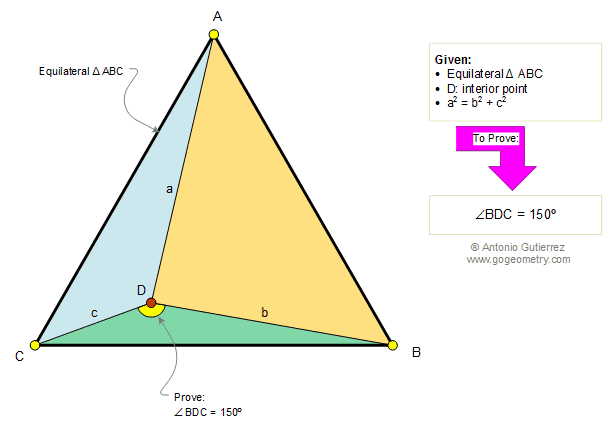# Problem 101. Equilateral Triangle, Pythagoras, Angles. High School, College

 In the diagram below, given an equilateral triangle ABC, D is an interior point. If a2 = b2 +c2 prove that the measure of angle BDC is equal to 150°. View or post a solution. .HINTS:

• Pythagorean Theorem Converse

• Congruence of Triangles SAS

• The sum of the measures of the three angles of a triangle is 180.

Home | Geometry | Search | Problems | Congruence | Right Triangle | All Problems | 101-110 | Visual Index | Email | View or post a solution | by Antonio Gutierrez